# python写的串口助手并连接腾讯云服务器数据库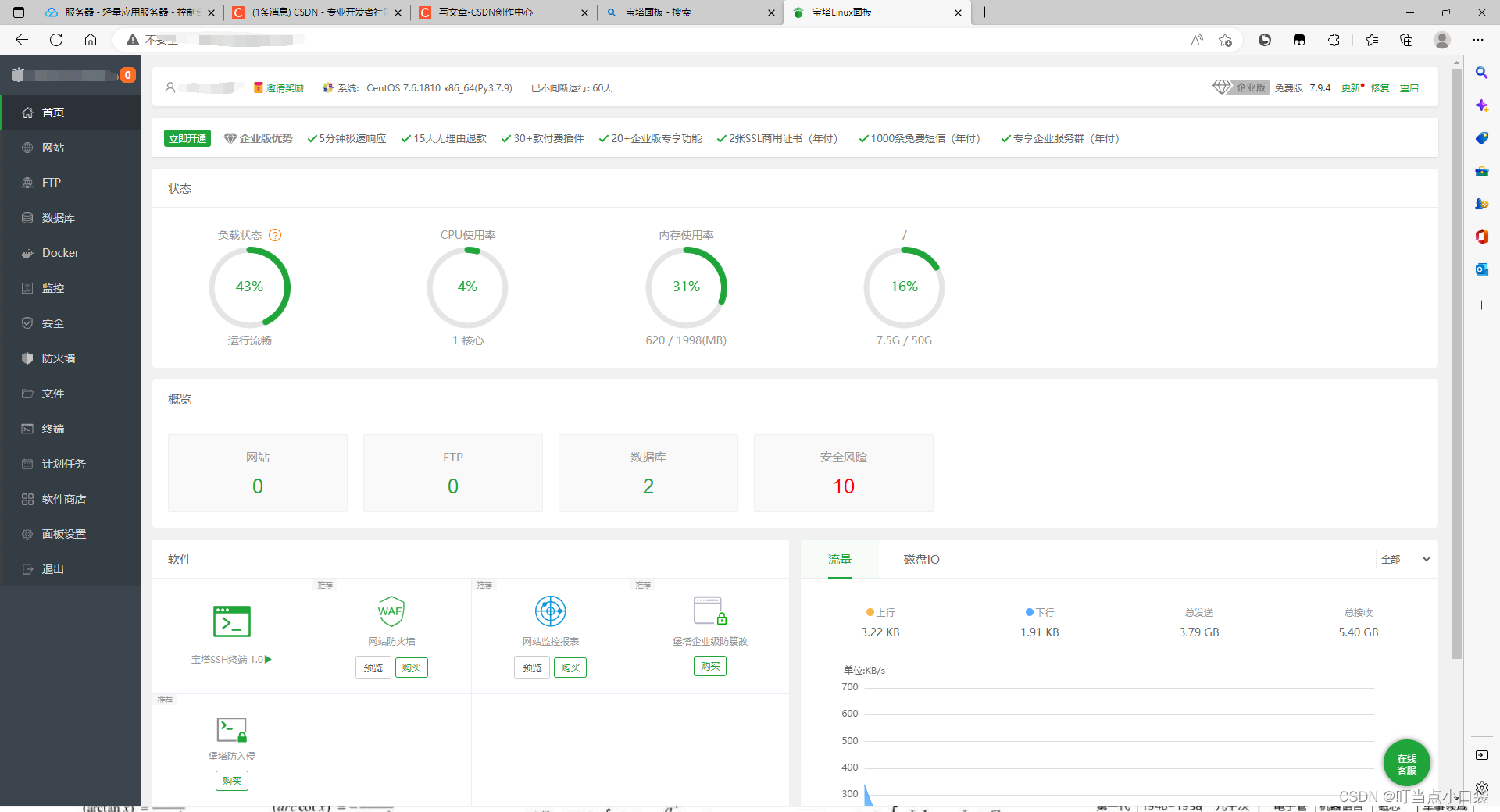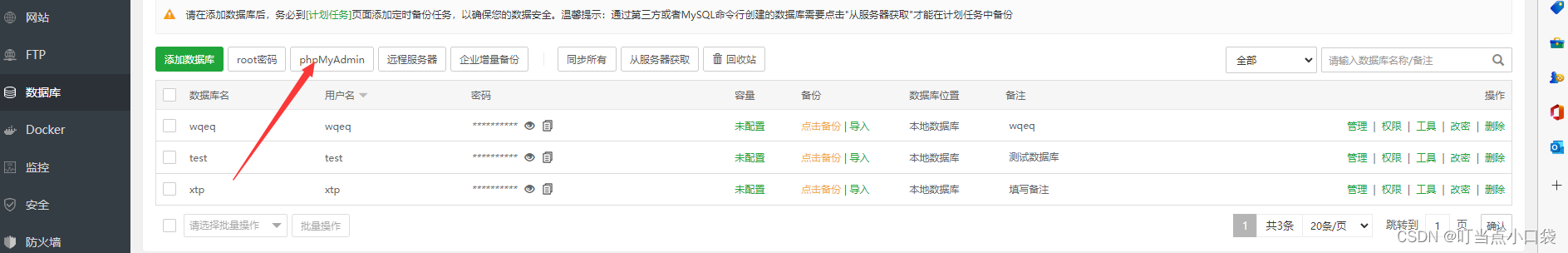进入后找到你创建的数据库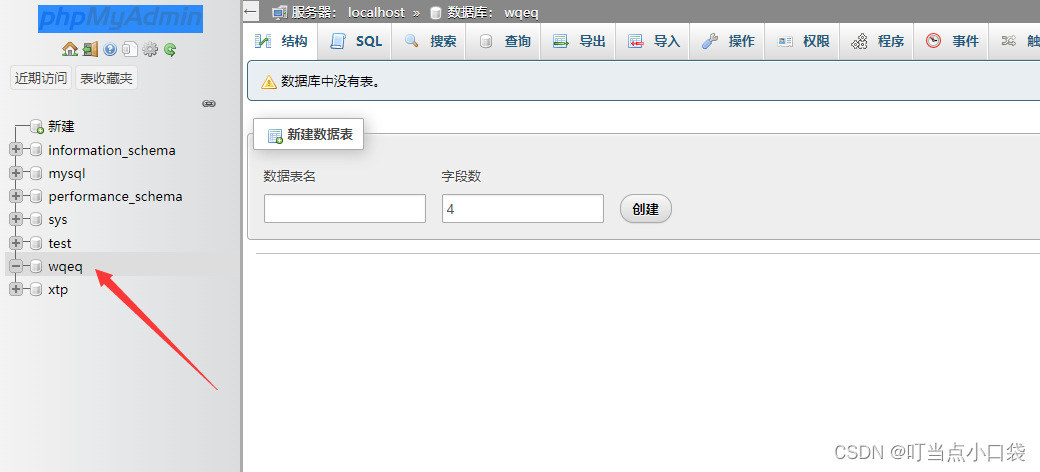输入自己想创建的数据表名，字段数代表有几个输入框，比如：账号、密码，那么字段数为2。点击创建即可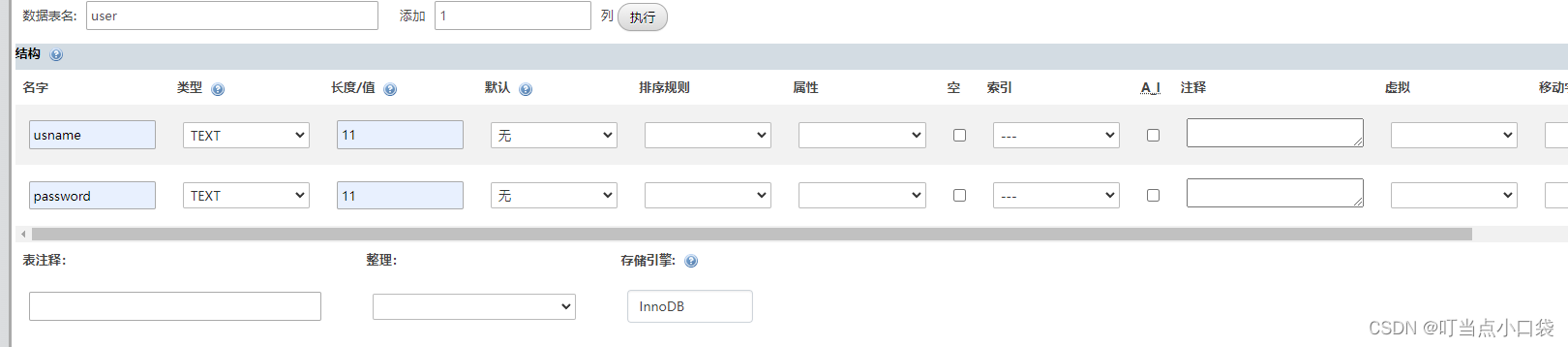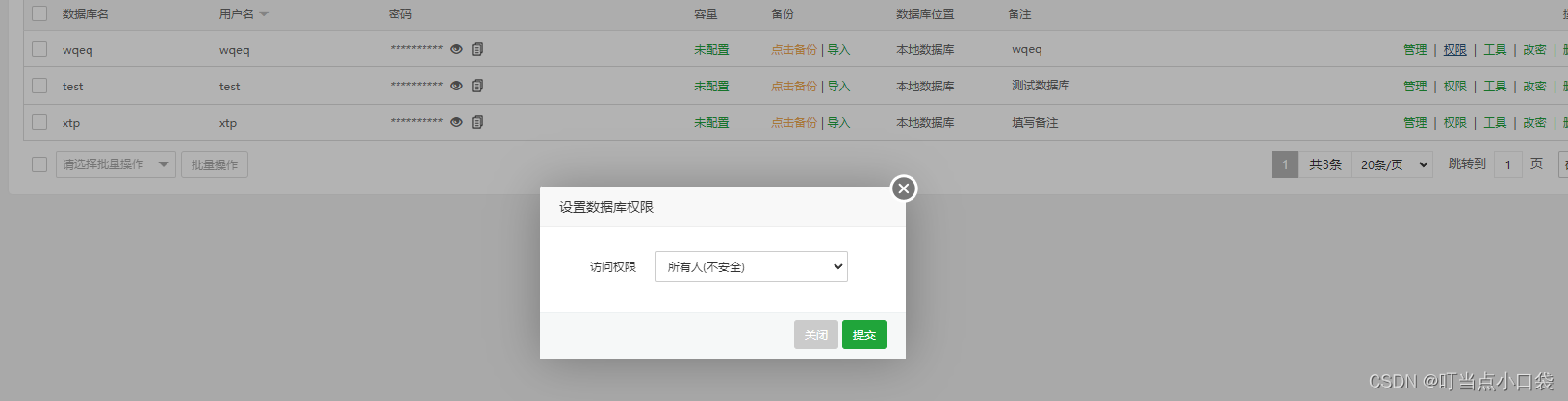我们再看Python连接服务器的代码。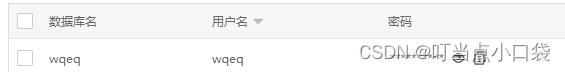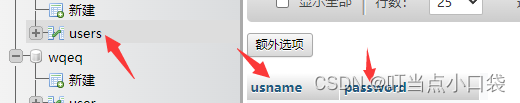需要填写的内容都在这

    def go_to_login(self):
uname = self.ui.lineEdit_4.text()
upwd = self.ui.lineEdit_3.text()
#               db='test', port=3306, charset='utf8')
码', db='数据库名', port=3306, charset='utf8')
#  其他的保持不变
#  得到一个游标对象
cs1 = conn.cursor()
# 2, 执行sql语句
sql = """select password(创建的列名) from users(创建的表名) where usname(创建的列名) =%s """
cs1.execute(sql, (uname,))
result = cs1.fetchone()

if result == None:
self.ui.label_7.setText("用户名错误，登录失败")
elif result == upwd:
homepageWindow()
self.close()
else:
self.ui.label_7.setText("密码错误，登录失败")


    def go_to_login(self):
uname = self.ui.lineEdit_4.text()
upwd = self.ui.lineEdit_3.text()
db='test', port=3306, charset='utf8')
#  得到一个游标对象
cs1 = conn.cursor()
# 2, 执行sql语句
sql = """select password from users where usname =%s """
cs1.execute(sql, (uname,))
result = cs1.fetchone()

if result == None:
self.ui.label_7.setText("用户名错误，登录失败")
elif result == upwd:
homepageWindow()
self.close()
else:
self.ui.label_7.setText("密码错误，登录失败")

def go_to_enroll(self):
uname = self.ui.lineEdit_5.text()
upwd = self.ui.lineEdit_6.text()

# 打开与数据库的连接
db='test', port=3306, charset='utf8')
cur = conn.cursor()
# 判断用户名是否存在
sql = 'select count(*) from users where usname=%s'
# params = [uname]
cur.execute(sql, (uname,))
result = cur.fetchone()
if result:
self.ui.label_8.setText('用户名已经存在，请重新注册')
else:
# 用户名不存在
sql = 'insert into users(usname, password) values(%s, %s)'
# params = [uname, upwd]
result = cur.execute(sql, (uname, upwd))
conn.commit()
if result == 1:
self.ui.label_8.setText('注册成功')
else:
self.ui.label_8.setText('注册失败')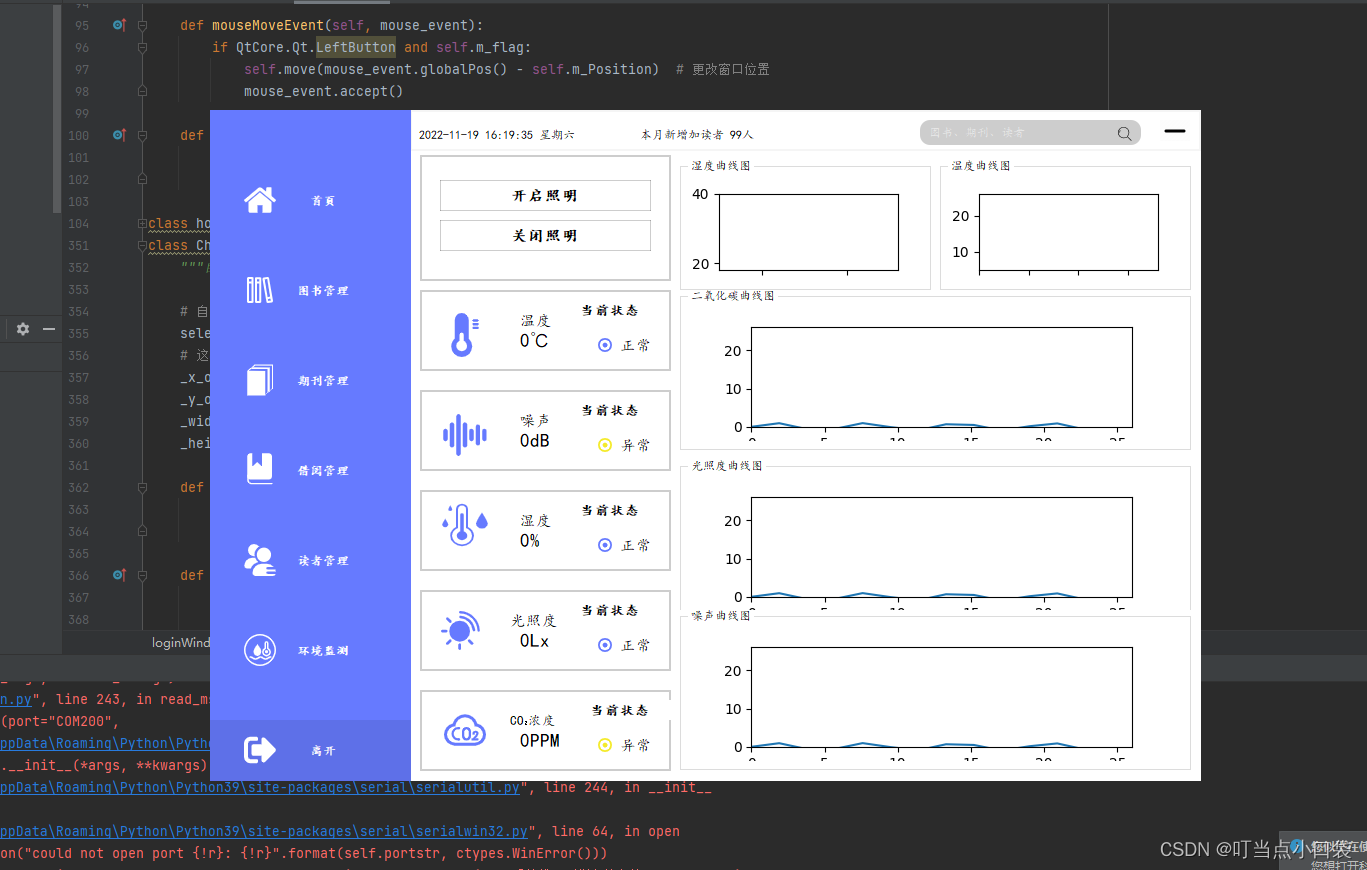因为采集的信息是温湿度之类的，所有自己也想做一个用matplotlib库的数据可视化，但是确定没有太多的时间就没有深入的去研究，只加入了库，没有设置变量这些。做一个串口助手，重要的地方在，接收信息并处理，发送16进制信息等。接收信息处理等。如何提取数据有效需要实际操作，因为我们是接收stm32的数据，所以用ser.read(100)来处理，ser为一个类，9代表一次读取多少位的数据，如这串FF F5 05 02 01 00 00 01 03数据。如果发送数据则使用b=bytearray([0xFF,0xF5,0x05,0x02,0x01,0x00,0x00,0x01,0x03])
b=bytes.fromhex('FF F5 05 02 01 00 00 01 03')
ser.write(d)

    def read_msg_thring(self):
t.start()

# 接收数据

global ser
ser = serial.Serial(port="COM200",
baudrate=38200,
bytesize=serial.SEVENBITS,
parity=serial.PARITY_NONE,
stopbits=serial.STOPBITS_TWO,
timeout=0.5)
print("开启成功")
while True:
if ((data == 0xFF) & (data == 0xFD)):
if (data == 0x00):
x=float(int(chr(data))+(int(chr(data))/10))
# y=float(x * 1000 / 3.3)
self.ui.label_135.setText((str(x) + '0Lx'))

if (data == 0x01):
self.ui.label_99.setText((str(int(data)+(int(data))/10))+"℃")
self.ui.label_104.setText((str(int(data) +(int(data))/10))+'%')

def kaiq(self):
# data = bytearray([0xFF,0xF5,0x05,0x02,0x01,0x00,0x00,0x01,0x03])
# ser.write(data)
d = bytes.fromhex('FF F5 05 02 01 00 00 01 03')
ser.write(d)
# ser.write(b'\xFA\xFB\x07\x01\x00\x00\x00\x00\x00')
print("打开")
# FF F5 05 02 01 00 00 01 03
def guan(self):
data = bytearray([0xFF,0xF5,0x05,0x02,0x01,0x00,0x00,0x02,0x02])
ser.write(data)被折叠的  条评论 为什么被折叠?到【灌水乐园】发言¥2 ¥4 ¥6 ¥10 ¥20余额支付 (余额：-- )扫码支付获取中扫码支付点击重新获取扫码支付1.余额是钱包充值的虚拟货币，按照1:1的比例进行支付金额的抵扣。
2.余额无法直接购买下载，可以购买VIP、C币套餐、付费专栏及课程。余额充值